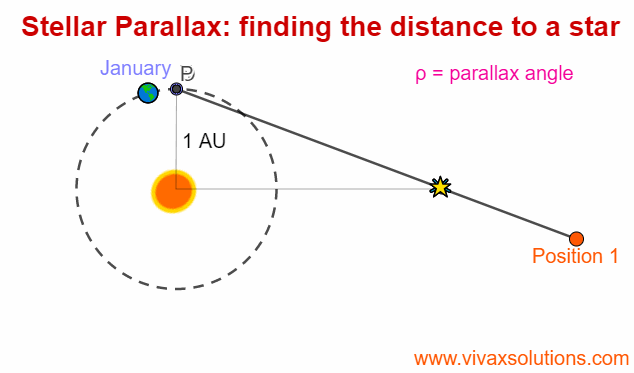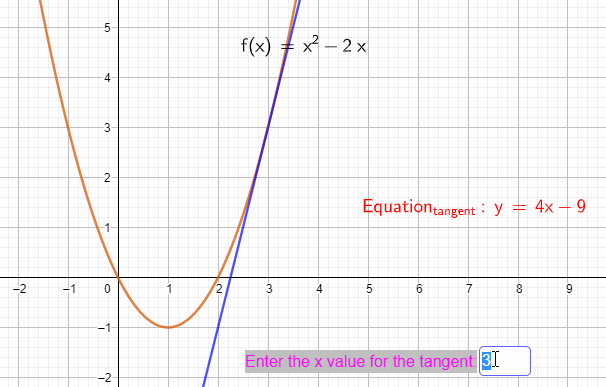## Posts

Showing posts from February 20, 2022

### Terminal Velocity - A Level PhysicsWhen an object comes down under gravity in the air, it is subjected at least two forces, the weight - W  -  and the drag / air resistance - R.  The resultant force, F = W - R F = ma  W - R = ma, where m and a are the mass of the object and the drag respectively. As the object comes down, the drag, goes up as the latter is proportional to the speed at a given time. That means the acceleration goes down as well. The slowing gradient of the above curve reflects that.      At a certain point, R becomes equal to W and the resultant force is zero. That means, the acceleration becomes zero. The object in question now moves with a constant speed. It is called the terminal velocity. This can be shown in a lab by dropping a lead ball into a tall cylinder that contains a viscous fluid such as glycerine.  When the ball moves through the same distance in equal times, it indicates the ball has reached its terminal velocity.  You can practise it here interactively:

### Stellar Parallax for A Level Physics - astrophysicsParallax is an optical phenomenon when the apparent position of an an object changes with the position of the observer. In order to experience this, you can hold the your index finger some distance from your right eye - or left eye, depending on your convenience - and then moving your head slowly to the left  or right, while focusing on the finger against the background. You may notice that your finger goes to the left when the head moves to the right and vice versa. This is parallax. The phenomenon can be used to find the distance to a far away star. In this case, the finger represents the star and the background, of course, is space. Since the parallax angle measured will be so small if we choose two points on the Earth, even if they are poles apart, physicist have decided to use the orbit of the Earth around the Sun for the difficult measurement.  In order to achieve that, they choose two points on the orbit that are diametrically opposite - with 1 AU apart: the apparent position

### Basic Calculus - differentiation for beginners IGCSE, AS, A-LevelThe process of finding the gradient or slope of a function is the differentiation. We used to find the gradient of a straight line, just by dividing the change in 'y' by change in 'x', in a certain range of values. When it comes to a curve, however, it is not easy to find the gradient or slope as the very thing we want to measure, keeps changing from point to point. In the above applet, a tangent is drawn to find the gradient at a given point on the curve. With differentiation, it is just two steps away. E.g. Let y = x 2   Gradient of the curve at any point = dy/dx = 2x Gradient at x = 2, dy/dx = 2x2 = 4 Gradient at x = 0, dy/dx = 2x0 = 0 Gradient at x = -3, dy/dx = 2x-3 = -6 For a more detailed tutorial with lots of worked examples on the following, please use this link on the website connected to this blog. Finding differential coefficient from the first principles Finding the equations of a tangent and a normal to a curve Increasing and decreasing funct